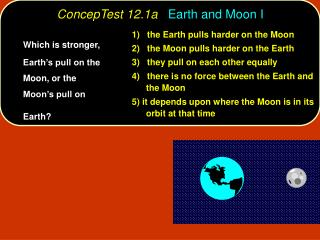# ConcepTest 12.1a Earth and Moon I - PowerPoint PPT PresentationDownload PresentationConcepTest 12.1a Earth and Moon I

ConcepTest 12.1a Earth and Moon IDownload Presentation## ConcepTest 12.1a Earth and Moon I

- - - - - - - - - - - - - - - - - - - - - - - - - - - E N D - - - - - - - - - - - - - - - - - - - - - - - - - - -
##### Presentation Transcript

1. ConcepTest 12.1aEarth and Moon I 1) the Earth pulls harder on the Moon 2) the Moon pulls harder on the Earth 3) they pull on each other equally 4) there is no force between the Earth and the Moon 5) it depends upon where the Moon is in its orbit at that time Which is stronger, Earth’s pull on the Moon, or the Moon’s pull on Earth?

2. ConcepTest 12.1aEarth and Moon I 1) the Earth pulls harder on the Moon 2) the Moon pulls harder on the Earth 3) they pull on each other equally 4) there is no force between the Earth and the Moon 5) it depends upon where the Moon is in its orbit at that time Which is stronger, Earth’s pull on the Moon, or the Moon’s pull on Earth? By Newton’s 3rd Law, the forces are equal and opposite.

3. ConcepTest 12.1bEarth and Moon II 1) one quarter 2) one half 3) the same 4) two times 5) four times If the distance to the Moon were doubled, then the force of attraction between Earth and the Moon would be:

4. ConcepTest 12.1bEarth and Moon II 1) one quarter 2) one half 3) the same 4) two times 5) four times If the distance to the Moon were doubled, then the force of attraction between Earth and the Moon would be: The gravitational force depends inversely on the distance squared. So if you increase the distance by a factor of 2, the force will decrease by a factor of 4. Follow-up: What distance would increase the force by a factor of 2?

5. ConcepTest 12.5In the Space Shuttle 1) They are so far from Earth that Earth’s gravity doesn’t act any more. 2) Gravity’s force pulling them inward is cancelled by the centripetal force pushing them outward. 3) While gravity is trying to pull them inward, they are trying to continue on a straight-line path. 4) Their weight is reduced in space so the force of gravity is much weaker. Astronauts in the space shuttle float because:

6. ConcepTest 12.5In the Space Shuttle 1) They are so far from Earth that Earth’s gravity doesn’t act any more. 2) Gravity’s force pulling them inward is cancelled by the centripetal force pushing them outward. 3) While gravity is trying to pull them inward, they are trying to continue on a straight-line path. 4) Their weight is reduced in space so the force of gravity is much weaker. Astronauts in the space shuttle float because: Astronauts in the space shuttle float because they are in “free fall” around Earth, just like a satellite or the Moon. Again, it is gravity that provides the centripetal force that keeps them in circular motion. Follow-up: How weak is the value of g at an altitude of 300 km?

7. ConcepTest 12.6Guess my Weight If you weigh yourself at the equator of Earth, would you get a bigger, smaller or similar value than if you weigh yourself at one of the poles? 1) bigger value 2) smaller value 3) same value

8. ConcepTest 12.6Guess my Weight If you weigh yourself at the equator of Earth, would you get a bigger, smaller or similar value than if you weigh yourself at one of the poles? 1) bigger value 2) smaller value 3) same value The weight that a scale reads is the normal force exerted by the floor (or the scale). At the equator, you are in circular motion, so there must be a net inward force toward Earth’s center. This means that the normal force must be slightly less than mg. So the scale would register something less than your actual weight.

9. 2d 2m 5 Earth 4 d 3 2 1 m ConcepTest 12.7Force Vectors A planet of mass m is a distance d from Earth. Another planet of mass 2m is a distance 2d from Earth. Which force vector best represents the direction of the total gravitation force on Earth?

10. 2d 2m 5 4 d 3 2 1 m ConcepTest 12.7Force Vectors A planet of mass m is a distance d from Earth. Another planet of mass 2m is a distance 2d from Earth. Which force vector best represents the direction of the total gravitation force on Earth? The force of gravity on the Earth due to m is greater than the force due to 2m, which means that the force component pointing down in the figure is greater than the component pointing to the right. F2m = GME(2m) / (2d)2 = 1/2 GMm / d2 Fm = GMEm / d2 = GMm / d2